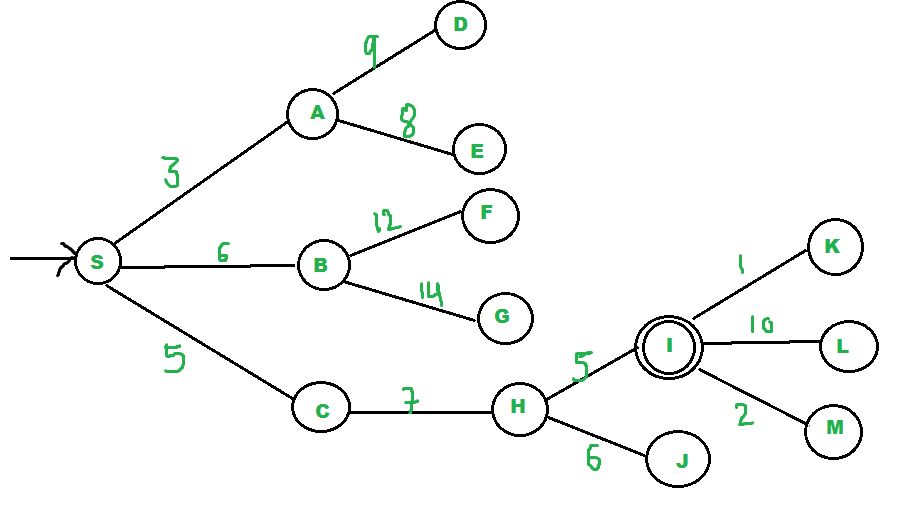# Best First Search (Informed Search)

• Difficulty Level : Easy
• Last Updated : 18 Jul, 2022

In BFS and DFS, when we are at a node, we can consider any of the adjacent as the next node. So both BFS and DFS blindly explore paths without considering any cost function.

The idea of Best First Search is to use an evaluation function to decide which adjacent is most promising and then explore.

Best First Search falls under the category of Heuristic Search or Informed Search.

Implementation of Best First Search:

We use a priority queue or heap to store the costs of nodes that have the lowest evaluation function value. So the implementation is a variation of BFS, we just need to change Queue to PriorityQueue.

```// Pseudocode for Best First Search
Best-First-Search(Graph g, Node start)
1) Create an empty PriorityQueue
PriorityQueue pq;
2) Insert "start" in pq.
pq.insert(start)
3) Until PriorityQueue is empty
u = PriorityQueue.DeleteMin
If u is the goal
Exit
Else
Foreach neighbor v of u
If v "Unvisited"
Mark v "Visited"
pq.insert(v)
Mark u "Examined"
End procedure```

Illustration:

Let us consider the below example:• We start from source “S” and search for goal “I” using given costs and Best First search.

• pq initially contains S
• We remove s from and process unvisited neighbors of S to pq.
• pq now contains {A, C, B} (C is put before B because C has lesser cost)

• We remove A from pq and process unvisited neighbors of A to pq.
• pq now contains {C, B, E, D}

• We remove C from pq and process unvisited neighbors of C to pq.
• pq now contains {B, H, E, D}

• We remove B from pq and process unvisited neighbors of B to pq.
• pq now contains {H, E, D, F, G}
• We remove H from pq.
• Since our goal “I” is a neighbor of H, we return.

Below is the implementation of the above idea:

## C++

 `// C++ program to implement Best First Search using priority``// queue``#include ``using` `namespace` `std;``typedef` `pair<``int``, ``int``> pi;` `vector > graph;` `// Function for adding edges to graph``void` `addedge(``int` `x, ``int` `y, ``int` `cost)``{``    ``graph[x].push_back(make_pair(cost, y));``    ``graph[y].push_back(make_pair(cost, x));``}` `// Function For Implementing Best First Search``// Gives output path having lowest cost``void` `best_first_search(``int` `actual_Src, ``int` `target, ``int` `n)``{``    ``vector<``bool``> visited(n, ``false``);``    ``// MIN HEAP priority queue``    ``priority_queue, greater > pq;``    ``// sorting in pq gets done by first value of pair``    ``pq.push(make_pair(0, actual_Src));``    ``int` `s = actual_Src;``    ``visited[s] = ``true``;``    ``while` `(!pq.empty()) {``        ``int` `x = pq.top().second;``        ``// Displaying the path having lowest cost``        ``cout << x << ``" "``;``        ``pq.pop();``        ``if` `(x == target)``            ``break``;` `        ``for` `(``int` `i = 0; i < graph[x].size(); i++) {``            ``if` `(!visited[graph[x][i].second]) {``                ``visited[graph[x][i].second] = ``true``;``                ``pq.push(make_pair(graph[x][i].first,graph[x][i].second));``            ``}``        ``}``    ``}``}` `// Driver code to test above methods``int` `main()``{``    ``// No. of Nodes``    ``int` `v = 14;``    ``graph.resize(v);` `    ``// The nodes shown in above example(by alphabets) are``    ``// implemented using integers addedge(x,y,cost);``    ``addedge(0, 1, 3);``    ``addedge(0, 2, 6);``    ``addedge(0, 3, 5);``    ``addedge(1, 4, 9);``    ``addedge(1, 5, 8);``    ``addedge(2, 6, 12);``    ``addedge(2, 7, 14);``    ``addedge(3, 8, 7);``    ``addedge(8, 9, 5);``    ``addedge(8, 10, 6);``    ``addedge(9, 11, 1);``    ``addedge(9, 12, 10);``    ``addedge(9, 13, 2);` `    ``int` `source = 0;``    ``int` `target = 9;` `    ``// Function call``    ``best_first_search(source, target, v);` `    ``return` `0;``}`

## Java

 `// Java program to implement Best First Search using priority``// queue``import` `java.util.ArrayList;``import` `java.util.PriorityQueue;` `class` `GFG``{``  ``static` `ArrayList > adj = ``new` `ArrayList<>();` `  ``// Function for adding edges to graph``  ``static` `class` `edge ``implements` `Comparable``  ``{``    ``int` `v, cost;``    ``edge(``int` `v, ``int` `cost)``    ``{``      ``this``.v = v;``      ``this``.cost = cost;``    ``}``    ``@Override` `public` `int` `compareTo(edge o)``    ``{``      ``if` `(o.cost < cost) {``        ``return` `1``;``      ``}``      ``else``        ``return` `-``1``;``    ``}``  ``}` `  ``public` `GFG(``int` `v)``  ``{``    ``for` `(``int` `i = ``0``; i < v; i++) {``      ``adj.add(``new` `ArrayList<>());``    ``}``  ``}` `  ``// Function For Implementing Best First Search``  ``// Gives output path having lowest cost``  ``static` `void` `best_first_search(``int` `source, ``int` `target, ``int` `v)``  ``{` `    ``// MIN HEAP priority queue``    ``PriorityQueue pq = ``new` `PriorityQueue<>();``    ``boolean` `visited[] = ``new` `boolean``[v];``    ``visited = ``true``;` `    ``// sorting in pq gets done by first value of pair``    ``pq.add(``new` `edge(source, -``1``));``    ``while` `(!pq.isEmpty()) {``      ``int` `x = pq.poll().v;` `      ``// Displaying the path having lowest cost``      ``System.out.print(x + ``" "``);``      ``if` `(target == x) {``        ``break``;``      ``}``      ``for` `(edge adjacentNodeEdge : adj.get(x)) {``        ``if` `(!visited[adjacentNodeEdge.v]) {``          ``visited[adjacentNodeEdge.v] = ``true``;``          ``pq.add(adjacentNodeEdge);``        ``}``      ``}``    ``}``  ``}``  ``void` `addedge(``int` `u, ``int` `v, ``int` `cost)``  ``{``    ``adj.get(u).add(``new` `edge(v, cost));``    ``adj.get(v).add(``new` `edge(u, cost));``  ``}` `  ``// Driver code to test above methods``  ``public` `static` `void` `main(String args[])``  ``{` `    ``// No. of Nodes``    ``int` `v = ``14``;` `    ``// The nodes shown in above example(by alphabets) are``    ``// implemented using integers addedge(x,y,cost);``    ``GFG graph = ``new` `GFG(v);``    ``graph.addedge(``0``, ``1``, ``3``);``    ``graph.addedge(``0``, ``2``, ``6``);``    ``graph.addedge(``0``, ``3``, ``5``);``    ``graph.addedge(``1``, ``4``, ``9``);``    ``graph.addedge(``1``, ``5``, ``8``);``    ``graph.addedge(``2``, ``6``, ``12``);``    ``graph.addedge(``2``, ``7``, ``14``);``    ``graph.addedge(``3``, ``8``, ``7``);``    ``graph.addedge(``8``, ``9``, ``5``);``    ``graph.addedge(``8``, ``10``, ``6``);``    ``graph.addedge(``9``, ``11``, ``1``);``    ``graph.addedge(``9``, ``12``, ``10``);``    ``graph.addedge(``9``, ``13``, ``2``);` `    ``int` `source = ``0``;``    ``int` `target = ``9``;` `    ``// Function call``    ``best_first_search(source, target, v);``  ``}``}` `// This code is contributed by Prithi_Dey`

## Python3

 `from` `queue ``import` `PriorityQueue``v ``=` `14``graph ``=` `[[] ``for` `i ``in` `range``(v)]` `# Function For Implementing Best First Search``# Gives output path having lowest cost`  `def` `best_first_search(actual_Src, target, n):``    ``visited ``=` `[``False``] ``*` `n``    ``pq ``=` `PriorityQueue()``    ``pq.put((``0``, actual_Src))``    ``visited[actual_Src] ``=` `True``    ` `    ``while` `pq.empty() ``=``=` `False``:``        ``u ``=` `pq.get()[``1``]``        ``# Displaying the path having lowest cost``        ``print``(u, end``=``" "``)``        ``if` `u ``=``=` `target:``            ``break` `        ``for` `v, c ``in` `graph[u]:``            ``if` `visited[v] ``=``=` `False``:``                ``visited[v] ``=` `True``                ``pq.put((c, v))``    ``print``()` `# Function for adding edges to graph`  `def` `addedge(x, y, cost):``    ``graph[x].append((y, cost))``    ``graph[y].append((x, cost))`  `# The nodes shown in above example(by alphabets) are``# implemented using integers addedge(x,y,cost);``addedge(``0``, ``1``, ``3``)``addedge(``0``, ``2``, ``6``)``addedge(``0``, ``3``, ``5``)``addedge(``1``, ``4``, ``9``)``addedge(``1``, ``5``, ``8``)``addedge(``2``, ``6``, ``12``)``addedge(``2``, ``7``, ``14``)``addedge(``3``, ``8``, ``7``)``addedge(``8``, ``9``, ``5``)``addedge(``8``, ``10``, ``6``)``addedge(``9``, ``11``, ``1``)``addedge(``9``, ``12``, ``10``)``addedge(``9``, ``13``, ``2``)` `source ``=` `0``target ``=` `9``best_first_search(source, target, v)` `# This code is contributed by Jyotheeswar Ganne`

Output

`0 1 3 2 8 9 `

Analysis :

• The worst-case time complexity for Best First Search is O(n * log n) where n is the number of nodes. In the worst case, we may have to visit all nodes before we reach goal. Note that priority queue is implemented using Min(or Max) Heap, and insert and remove operations take O(log n) time.
• The performance of the algorithm depends on how well the cost or evaluation function is designed.

Special cases of Best first search:

1. Greedy Best first search algorithm
2. A* search algorithm

This article is contributed by Shambhavi Singh. If you like GeeksforGeeks and would like to contribute, you can also write an article using write.geeksforgeeks.org or mail your article to review-team@geeksforgeeks.org. See your article appearing on the GeeksforGeeks main page and help other Geeks.

My Personal Notes arrow_drop_up